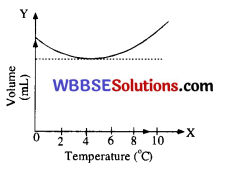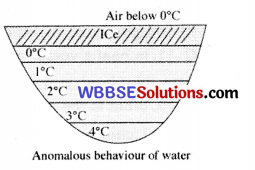# WBBSE Class 9 Physical Science Notes Chapter 6 Heat

Comprehensive WBBSE Class 9 Physical Science Notes Chapter 6 Heat can help students make connections between concepts.

## Heat Class 9 WBBSE Notes

Heat : It is an external agency whose absorption turns a body hot and extraction turns a body cold.

Sensible heat : It is the type of heat that is perceptible by senses. This causes rise of temperature of a body that can be measured with a thermometer.

Latent heat : It is the quantity of heat absorbed or released when a substance of unit mass undergoes a physical change of state at a constant temperature and pressure.

Radiant heat : It transmits from a source of heat in all directions with the speed of light and in the form of waves.Temperature : The temperature of a body is the thermal condition which determines whether it will absorb heat from other body or release heat to that body when they are kept in contact.

Lower fixed point of a thermometer : At normal atmospheric pressure, the temperature at which ice melts into water or pure water freezes into ice is called the lower fixed point of a thermometer.

Upper fixed point of a thermometer : It is the temperature at which pure water boils and transforms into steam under normal atmospheric pressure.

Fundamental interval : The range of temperature between the upper and lower fixed points is called fundamental interval.

Celsius scale of temperature : In this scale, the lower fixed point is 0°C and the upper fixed point is 100°C. The fundamental difference is divided into hundred equal parts and each part represents 1°C.

Fahrenheit scale of temperature : In this scale, the lower fixed point is 32°F and the upper fixed point is 212°F. The fundamental difference is divided into 180 equal parts and each part represents 1°F.

Kelvin scale of temperature : In this scale the lower fixed point is 273K and the upper fixed point is 373K, the fundamental interval is divided into 100 equal divisions like that in the Celsius scale. Each division represents 1K.Calorie : It is the amount of heat required to raise the temperature of one gram of pure water through 1°C.

Specific heat : It is defined as the quantity of heat required to raise the temperature of unit mass of it by 1 degree.

Specific heat capacity of a substance : Specific heat capacity of a substance is the quantity of heat required to raise the temperature of 1kg of the substance through 1K.

Units of specific heat :

• CGS : calg-10 C-1
• SI : Jkg-1K-1

Thermal capacity or heat capacity of a body : It is defined as the quantity of heat needed to raise the temperature of the body for unit degree rise of temperature.

Units of heat capacity :

• CGS : calg-10 C-1
• SI : Jk-1

Water equivalent : The water equivalent of a body is the mass of water which will be heated through one degree by the amount of heat required to raise the temperature of the body through one degree.Units of water equivalent:

• CGS: gram (g)
• SI: kilogram (kg)

Fundamental principle of calorimetry :

Conditions :

• During the process of heat transfer, there is no heat exchange with the surrounding.
• No chemical reaction takes place between the bodies.

Principle :
Heat lost by the hotter body = Heat gained by the colder body.

First law of thermodynamics : Whenever mechanical work is converted into heat energy, the heat so obtained is directly proportional to the work done.
If, ‘W’ be the mechanical work done and ‘H’ be the corresponding heat energy produced, then from first law of thermodynamics,
W α H or W = JH
where J is the constant of proportionality and is known as mechanical equivalent of heat.

Mechanical equivalent of heat : The mechanical equivalent of heat is numerically equal to the work done to produce unit quantity of heat.

Unit of mechanical equivalent of heat :

• CGS: 4.8 x 107 erg cal-1
• SI : In SI, both heat and work are measured in Joule, so mechanical equivalent of heat has no unit and its value is 1.

Change of state : There are three states of matter – solid, liquid and gas. Substances can be changed from one state to other by application or removal of heat from them. This process is known as change of state.Mathematical expression of Latent heat : Q = mL
where Q is the amount of heat supplied (or extracted) to change the state of ‘m’ mass of substance and L is latent heat

Unit of Latent heat :

• CGS : cal g-1
• SI : J kg-1

Types of Latent heat:

• Latent heat of fusion
• Latent heat of solidification
• Latent heat of vaporisation
• Latent heat of condensation

Latent heat of fusion of ice and latent heat of solidification of water are both equal:

• CGS : 80 cal g-1
• SI: 336 × 105 Kg-1

Latent heat of vaporisation of water and latent heat of condensation of steam are both equal:

• CGS: 537cal g-1
• SI : 226 × 106 Jkg-1

Melting point : The melting point of a solid is the definite temperature at which it melts on heating under normal atmospheric pressure and the temperature remains constant until the melting is
completed.

Boiling point : The boiling point of a liquid is the temperature at which a liquid boils under normal atmospheric pressure. The boiling point of a liquid depends upon:

• The nature of the liquid
• Presence of dissolved substance
• The superincumbent pressure

pressure: Whenever a liquid evaporates at any temperature, the vapour exerts a definite pressure on everything in contact. This pressure is called vapour pressure of the liquid at that temperature.

Saturated vapour : If a liquid is allowed to evaporate in a closed space, after some time it is found that evaporation stops i.e. at a given temperature. there is a maximum limit to the amount of
vapour the space can hold. The space is said to be then saturated with vapour and the vapour is then called saturated vapour.

Unsaturated vapour : If at a given temperature, a space contains a smaller amount of vapour than the maximum possible amount that the space can hold, the space is then said to be unsaturated and the vapour is called unsaturated vapour.

Saturated vapour does not obey Charles’ law or Boyle’s law, while unsaturated vapour obeys charles’ and Boyle’s law.Critical temperature : For every substance in gaseous state there is a certain temperature such that if the substance is below this temperature, it can be liquefied by the application of suitable pressure,
and above this temperature, it cannot be liquefied, however large the pressure may be, then the temperature is called the critical temperature for that substance.

Gas : A substance at a temperature above critical temperature is called gas.

Vapour : When the substance is at a temperature below its critical temperature it is called vapour.

Anomalous expansion of water: Usually liquids expand on heating. But in the case of water we find deviation from this general behaviour of the liquids within a certain range of temperature. It is found that if certain mass of water at an temperature about 10°C be taken and allowed to cool, its volume is observed to fall gradually till it reaches the temperature of 4°C, when with further cooling the volume of water will increase instead of decreasing.The behaviour of water is some how different from other liquids. The volume of water is minimum at 4°C and its density is maximum at 4°C. This phenomenon is called the anomalous expansion of water. This phenomenon is called the Ienipentturi anomalous expansion of water.

Effect on marine life : Anomalous expansion of water has great practical importance on the marine life in the cold countries. In these countries when air above the surface of water of pond falls below 0°C, the water on the surface on cooling, become denser than that below and gradually sinks downwards.This continues until the temperature of water falls to 4°C. As the surface temperature falls further it becomes less dense than the water below, which is at 4°C and is the densest. It thus remains at the top, cooling C more and more and ultimately a layer of ice is formed there and remains there as ice is lighter than water. The layer of ice forms a poor conducting layer on the top, which prevents the heat from water below to pass into colder atmosphere above.The temperature of the deeper layers of the water in the pond remains nearly at 4°C and falls gradually to 0°C upwards till the layer of ice is reached. The marine life in water is thus saved.